# MARKOV CHAIN MONTE CARLO TECHNIQUES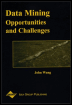Chapter XI - Bayesian Data Mining and Knowledge Discovery Data Mining: Opportunities and Challenges by John Wang (ed) Idea Group Publishing 2003Brought to you by Team-Fly

Markov Chain Monte Carlo (MCMC) techniques have been mainly responsible for the current momentum gained by Bayesian methods, since its application has enabled the use of A vast range of Bayesian models that had been previously deemed as intractable. With complicated models, it is rare that the posterior distribution can be computed directly. The simulation techniques described in the previous section are only adequate when dealing with low-dimensional problems but cannot be successfully applied in many real-world problems. This is where MCMC excels. MCMC is intrinsically a set of techniques for simulating from multivariate distributions. In order to introduce the topic, we need to provide some definitions regarding stochastic processes and Markov chains.

### Markov Chains

A random or stochastic process is a family of random variables {X(t), tT} defined over a given probability space and indexed by a parameter t that varies over an index set T. The values assumed by X(t) are called states. If T is discrete then the process is called a discrete-time process and is denoted as {Xn, n =1,2,..,n}. X1 is the initial state of the process and Xn is the state of the process at time n.

A Markov chain is a special case of a discrete-time process in which the following rule applies: At any given time n, the probabilities of the future state n+1 depend only on the current state Xn. This is expressed as: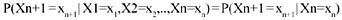In other words, if we generate a sequence of random variables X1, X2,.., Xn, such that the next state Xn+1 is a sample from a distribution that depends only on the current state of the chain, Xn, and does not depend on the rest of the chain X1, X2,..Xn-1, this sequence is called a Markov chain.

The conditional probability P(Xn+1 Xn) is known as the transition kernel of the Markov chain. When the transition kernel is independent of n, then it is said to be stationary, and the process is referred to as a time-homogeneous Markov chain. A finite Markov chain is such that there are only a finite number k of possible states s1,s2,..,sk, and the process must be in one of these k states. If any of these states can be reached from any other state in a finite number of moves, the chain is said to be irreducible.

### Markov Chain Simulation

The idea of Markov Chain simulation is to simulate a random walk in the parameter space that converges to a stationary distribution that is the target multivariate distribution π(x) that we want to simulate (typically a joint posterior distribution). It is possible to construct a Markov chain such that this stationary distribution is the target distribution π(x). Therefore, over time the draws Xn will look more and more like dependent samples from the target distribution. After hundreds of iterations, the chain will gradually forget the starting position X1, and it will gradually converge to the stationary distribution.

Several methods have been developed for constructing and sampling from transition distributions. Among them, the Metropolis algorithm and the Gibbs sampler are among the most powerful and popular methods currently in use. For a more complete description, see Gelman et al. (1995) and Neal (1993). Also, a complete set of lectures on this topic can be found in Rodriguez (1999).

### The Metropolis Algorithm

The original algorithm was developed by Metropolis in 1953 with the purpose of simulating the evolution of a system in a heat bath towards thermal equilibrium. In its rather recent application to statistics, the Metropolis algorithm creates a sequence of points (X1, X2, ) whose distributions converge to the target distribution π(x). Let q(y x) be the jumping (proposal) distribution. In the Metropolis algorithm, this jumping distribution must be symmetric, that is, q(xy) = q(yx) for all X,Y, and n. The algorithm proceeds as follows:

1. Given the current position Xn, the next candidate state is chosen by sampling a point Y from the proposal distribution q(yx)

2. The ratio of the densities is calculated as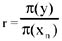3. Calculate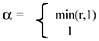4. With probability α accept the candidate value and set Xn+1 = Y; otherwise reject Y and set Xn+1 = Xn

5. Go to step 1

It can be proved that for a random walk on any proper distribution with positive probability of eventually jumping from a state to any other state, the Markov chain will have a unique stationary distribution. It can also be shown that the target distribution is the stationary distribution of the Markov chain generated by the Metropolis algorithm (see Gelman & Gelman, 1995).

 Brought to you by Team-Fly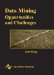Data Mining: Opportunities and Challenges
ISBN: 1591400511
EAN: 2147483647
Year: 2003
Pages: 194
Authors: John Wang